#从RNN到LSTM，到Attention

## 概述

RNN->LSTM->Attention->Tensorflow实践

RNN经典论文，虽然还是看着费劲，先mark一下，放在这里，以便日后阅读： Recurrent neural network based language model RNN用在语言模型上的开山之作.

## RNN

DNN不是挺好的嘛，干嘛还要RNN呢？处理时序问题！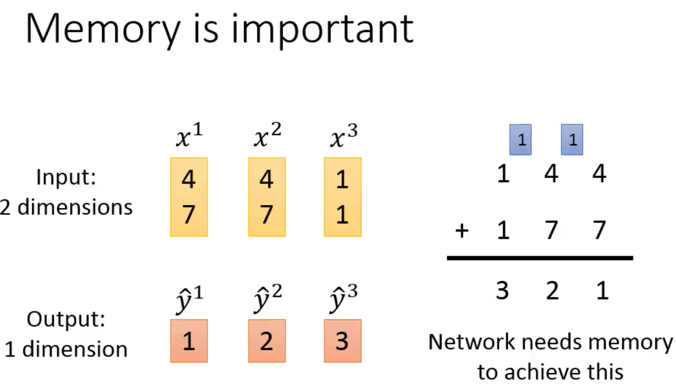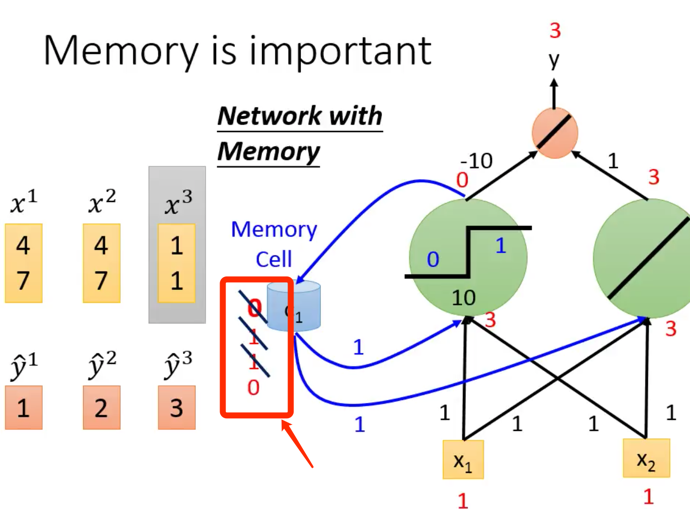DNN会对数据进行shuffle，说白了，我有x1,x2,x3…xn个数据，你灌谁输入都行，我期待的y也是独立的，但是，RNN不是，x1，x2，x3…xn之间就必须按照一个顺序来，不能颠倒，这样你预测出来的y序列也是有顺序的。注意x1-xn都是向量。 以翻译为例子可能比较好理解，每个中文，对应每个英文，每个中文词，其实都是一个独立的数据，对应为$x_i$，他本身就是个向量，比如使用word2vec词向量，这一对的词向量，就需要按照顺序对应，然后才能输出对应的一个英文的词向量输出，也就是翻译结果。 RNN是一个句子都输入完才是一个整个输入，也就是说，书的多个数据，必须打包在一起，按照严格的顺序，一起灌入。 我过去曾经有错觉，以为整句话是一个输入数据呢，自己还意淫出一个“句向量”的概念。

• 是一个数，对应到多维就是一个向量
• 这个向量是变的，会被更新，之前的那个就被丢弃了
• 每次的y输出其实都要打包收集起来，最终这个y序列是最终的输出结果

RNN的memory就是个向量。对，是向量！我还以为这个memory多大呢？！原来就是个向量，大失所望啊，呵呵。那么这个向量多少维度呢？好问题。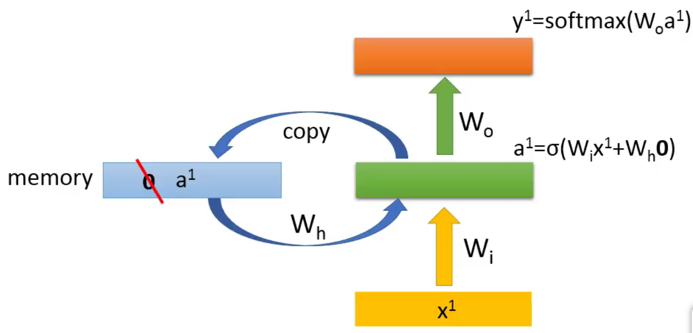a1=$\sigma$(Wix+ Whm)

• Wi是一个隐藏层的权重矩阵，
• Wh是memory向量对应的权重矩阵，
• m是记忆向量

y1=$softmax$(Wo*a1)

• Wo是上一个$softmax$的权重矩阵
• a1要被copy到memory向量里面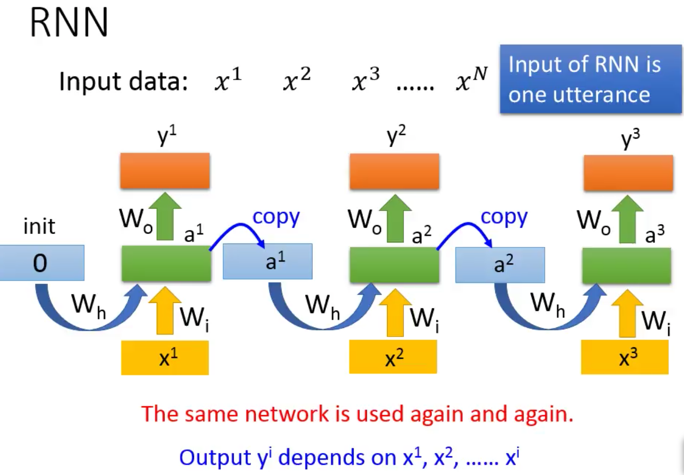[ 适用范围 ]

RNN都解决哪些类的问题呢？

• 比如N:N: 严格1对1，如POS词性标准、实体识别、信息提取
• 比如N:1，比如文章的情感识别，正面文章还是负面文章，就是1个判断结果
• 比如1:N，比如图片的看图说话，输入是一个图片的所有的像素组成的一个向量，输出是一堆的文字向量
• 比如M:N，如seq2seq，不再是经典RNN的每个字/词都要对着，而是M:N的关系，可多可少，不过没听懂他说何时断掉，因为他的那个例子“machine learning”-> “机器学习习惯好…”后面的序列可以一直产生下去呀（因为不是1对1嘛），李宏毅老师说，要给翻译结果一个中断符，不过怎么给，没听明白？？？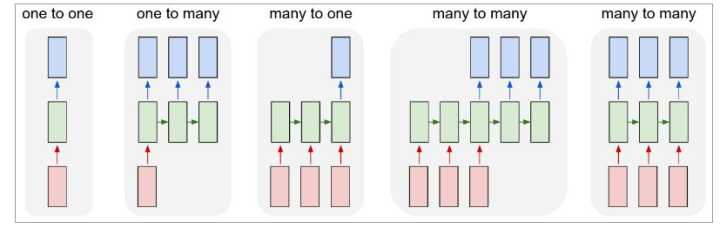[ 损失函数 ]

这个是李宏毅老师的损失函数（均方误差）这个是交叉熵的损失函数

$L(y,\hat y) = \frac{1}{N} \sum_{n \in N} y_n log\hat y_n$

• 第一个时刻$t1$，输入为“i”，预期（也就是样本输出数据提供的）是“我”，RNN模型根据这个信息计算出$s_1$，不过RNN最后还要softmax跑出来输出了一个词表向量，可能会在“我”这个维度上值稍微大一些，当然也可以小。这样，这个标签数据和输出数据就产生了差，这个差未来就可以用作反向调整所有的权值。 （样本输出数据为“我”，对应概率向量就是在“我”这个词的维度上为1，其余都是0）
• 第二个时刻$t2$，这个时候输入是“love”，对于RNN来说，这个时候，输出的softmax就依赖于两个输入啦，一个是新输入的“love”，还有是上一个时刻的输出的中间量$s_1$，两者共同作用，才得到了$s_2$

(s是中间结果，做softmax之前，下面图有说明)

• 然后是第三个“北京”，依赖于输入的“beijing”和上一个状态输出值$s_2$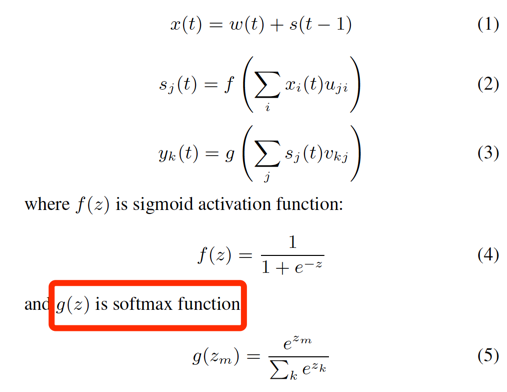$L(y,\hat y) = \frac{1}{N} \sum_{n \in N} log\hat y_n$

[ 训练过程&BPTT ]

RNN训练和DNN的BP不同的是，他的W要反复被更新，这个是因为某个$y_i$需要依赖于$a_i$，$a_i$又依赖于前面的$a_{i-1}$，就依赖于之前的$w_{i-1}$,….，然后递归向前。 w其实只有一份，所以每次更新的时候（就是每一层），都会更新这个w，这也是就是大家常说的，RNN的参数w是共享的。 举个例子，$y_3$是由$a_3$决定的，而$a_3$是由$x_3$和$a_2$一起决定的，而$a_2$是由$x_2$和$a_1$一起决定的，最后，$a_1$是由$x_1$决定的，这样，一个$y_3$的结果，实际上是由$x_1,x_2,x_3$一起决定的，感受到了吧。 这种不停往前倒腾的方法，就被起了个名字叫BPTT（back propagation through time）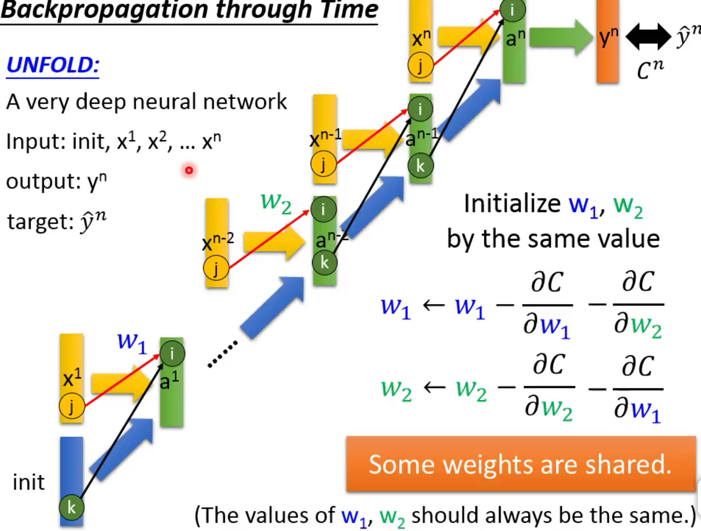BPTT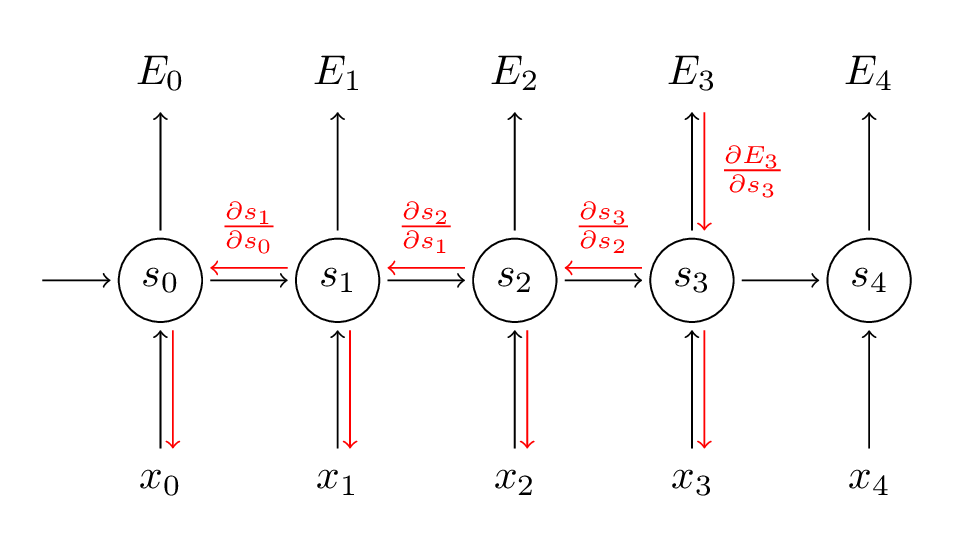$E_t= log(\hat y_t)$

$\hat y_t=softmax(s_t)$

$s_t=\sigma(a_t)$

$a_t = w_i x_t + w_h s_{t-1}$

• w_i是输入层的参数
• w_h是隐层参数，也就是和上一时刻的$s_{t-1}$相乘的参数

RNN的训练不好训练，狠困难，据说是“the error surface is rough”，就是他的cost函数不是平滑的凸函数，而是崎岖或者陡峭，不好训练。RNN的Training没有特别好的方法，只能有一些最佳实践方法： NAG，RMSProp，没有详细关注，未来用到再说。

## LSTM

LSTM要解决RNN的啥问题？[ 三重门 ]

LSTM，3个gate（门）

• 一个管是不是改把上次的输出输入到memory cell里：输入门
• 一个管是不是把memory cell的值吐给下一次序列：输出门
• 一个管是不是把memory cell里面的记忆之清空：忘记门

李宏毅老师版本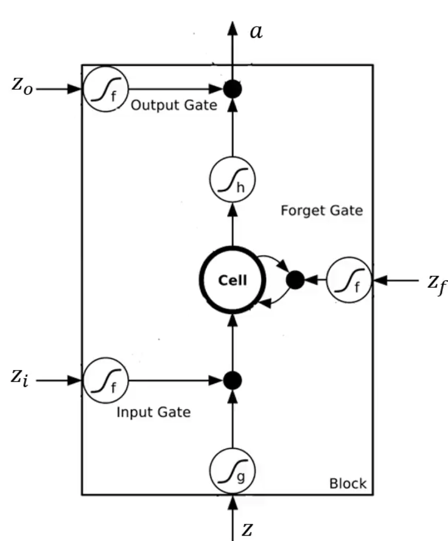LSTM一般版本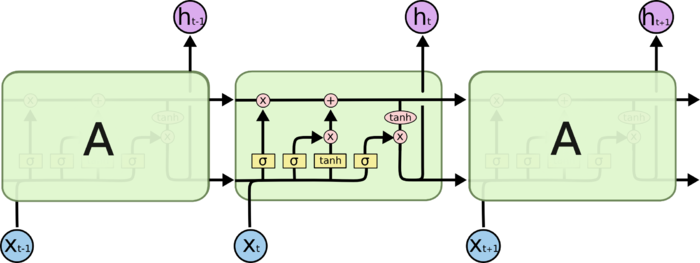LSTM一般版本就复杂了一些，李宏毅老师提过，就是最终上图中的a，实际上在生产版本中，也会把上一层的输出$C_{t-1}, h_{t-1}$，合并到下一层的输入中，继续使用。啥叫合并，就是把输入$x_i$和$h_{t-1}$俩向量做一个并，变成更大维度的一个向量，灌入。

[ 4个步骤 ]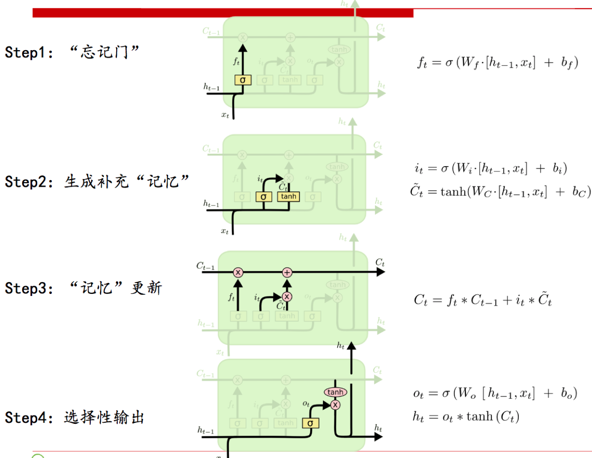• Step1 忘记门，你看它输出是一个是否忘记的概率，越接近0，越偏向于忘记。这个门是由什么决定的，上一次的输出$h_t$和本次的输入$x_t$，注意注意，是上次的输出$h_t$，而不是上次的$C_t-1$，诡异吧，论文就是这么设计的，不知道为何（不是我说的，July寒老师这么评论的） [$h_{t-1},x_t$]是把两个向量做了拼接。 对了，忘记啥东西啊？这点忘说了。忘记的是，上一次的状态，是不是让上一次的状态就过来了，然后和这一次的状态一起做个线性组合，变成下一次的状态，喂给下下次。
• Step2 准备新的信息，和信息通过的概率 $\bar C_t$就是生成新的信息，为何用tanh函数，据寒老师说，是为了BPTT的时候不至于梯度爆炸，不解？ $i_t$又是生成一个概率值，多大程度上接受这些新知识，所以，下一步就会看到 $\bar C_t$ * $i_t$ ，对了，这个式子很像忘记门啊，不过细看，不是一回事，对应的权值不一样，他是$W_f$，我是$W_i$，各玩各的，各为其主。
• Step3 得到最新的“记忆”内容，也就是$C_t$，他实际上是上一个$C_{t-1}$和刚才挑选出来的新信息$\bar C_t$，$C_{t-1}$乘以忘记概率$f_t$，$\bar C_{t}$乘以接受概率$i_t$，得到最终的本次要记忆下来的内容。 寒老师说，这个加法，可以避免RNN反向求导的时候，由于连乘导致的梯度消失的问题，不解？
• Step4 最后一步，输出的时候，再来一个概率判断$o_t$,才输出

LSTM变种很多，不过效果都大同小异。

[ 损失函数 ]

[ 如何训练 ]

[ 一些变种 ]

## Attentioni love beijing tian an men=>我爱北京天安门。Attention，实际上是两个RNN，输入的RNN，关注（Attention）+输出的RNN。

https://zhuanlan.zhihu.com/p/22081325

https://zhuanlan.zhihu.com/p/28054589